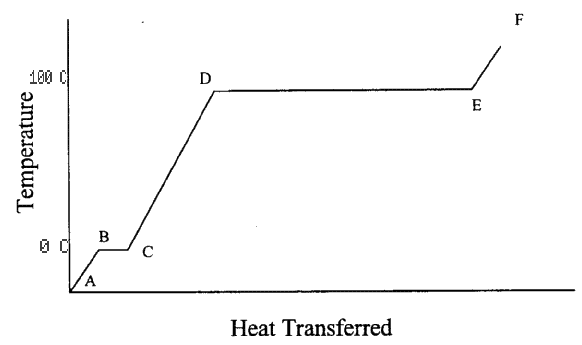### University of California, San Diego Physics 1b - Thermal Physics & Electromagnetism

 H. E. Smith Spring 2000Physics 1B - Tutorial #21. Very cold ice is placed in a closed container. Heat is then applied to the container. Below is a graph of the temperature inside the container plotted against the amount of heat delivered to the system.• Describe what is happening to the substance inside the container from point A to point F.
• A --> B ice temperature rising as heat enters container.
• B --> C ice is melting; heat --> heat of fusion necessary to melt ice. reached.
• C --> D water temperature is rising.
• D --> E water is evaporating; heat --> heat of vaporization.
• E --> F water vapor temperature is rising.

• Put temperature scales in Celsius on the vertical axis.
• B --> C = 0° C
• D --> E = 100° C
as above.

• If the heating rate is constant (dQ/dt = constant), compare the time it takes to heat from B to C to the time it takes to heat from D to E.

The latent heat of water vaporizing (heat of vaporization; Lv = 540 kcal/kg) is 6.75 times the latent heat of ice melting (heat of fusion; Lf = 80 kcal/kg), D --> E takes 6.75 times as long as the time from B --> C.

2. Materials A and B have equal densities. But A has twice the specific heat of B. You have two 100 g cubes made of materials A and B.
• Cube A, initially at 0°C, is placed in good thermal contact with cube B, initially at 100°C. Both are placed in a well-insulated container. What is the final temperature?

CA(100g)(T-0)=CB(100g)(100-T)
CA=2CB
2x(100g)(T-0)=(100g)(100-T) Solving: T=33.3 °C.

• Cubes A and B are both heated to 200°C, and then placed in room temperature environment. Which one cools faster?

The two cubes have the same surface area. Their heat loss rates are the same. Cube B has a lower heat capacity. Cube B cools faster.

3. A thermometer is laid out in direct sunlight. Does it measure the temperature of the air, of the sun, or of something else? The thermometer always measures the temperature of the thermometer. Its temperature is indicative of the temperature of another object only if it is in thermal equilibrium with the object (that's why your Mother makes you hold the thermometer under your tongue for so long - to establish thermal equilibrium. In principle, if the thermometer had perfect thermal radiative properties (called a blackbody) it would reach thermal equilbrium with the sun by radiative processes. Since the thermometer doesn't have perfect radiative properties (for one thing it would melt before reaching 6000K), in this case, its temperature is neither that of the sun nor that of the air.

4. The inside of an oven is at 400° F. But you can still put your hand in the oven as long as you don't touch anything. But since the air inside the oven is also at 400°F, why isn't your hand burned just the same? What if you leave your hand in the oven for a long time (e.g. 2 hours)?

Air is a very poor thermal conductor. (thousands of times less than that of iron). Therefore, its heat cannot be effectively transferred to your hand. If you touch the shelves made of iron, heat can be transferred quickly and you will be burned. If you leave your hand in the oven for too long, however, even just the air will transfer enough thermal energy to roast you.

5. While jogging, an average 65 kg student generates thermal energy at a rate of 300 W (about 0.4 horse power). To maintain a constant body temperature of 37°C, this energy has to be dissipated by perspiration or other mechanisms.
• If these mechanisms failed and the heat couldn't flow out of the student's body, for how long could a student run before irreversible body damage could occur? (Protein structures in the body are irreversibly damaged at 44°C or above. The specific heat capacity of a typical human body is 3480 J/kg.K, slightly less than that of water.)

At a heating rate of 300W, it takes

(3480J/kg/K)(65kg)(7K)/(300W)=5280s=1.5 hour

to raise the body temperature of the student by 7K, or 7 °C.

• If the heat loss is by sweat evaporation only, calculate the volume of water evaporated per minute (latent heat Lv = 539 cal/g). How many liters of water are needed to compensate the water loss of a two hour jog?

Latent heat Lv=539 cal/g=2.26 kJ/g. To dissipate at a rate of 300W, water has to evaporate at

(300W)/(2.26 kJ/g)=0.13g/s, or 0.13 ml per second, or 8 ml/min.

A 2-hour jog requires an intake of (8ml/min)(120min)=1 liter water.

• What other heat loss mechanisms do you think may be important for a runner?

The body looses heat due to breathing. Cold air is inhaled and warm air exhaled. The exhaled air is also more moist than the inhaled air. This water loss is actually part of the evaporated water calculated above. Heat is also lost due to conduction (from warm skin to cold air), and to convection as that warmed air rises and is replaced by cold air. Since the body is (usually) hotter than its surroundings more heat is lost due to radiation emitted to the surroundings than is gained from radiation absorbed from the surroundings.

Within the body heat is transferred mainly by blood flow. Blood is warmed as it passes through the heart and lungs, and cooled at the body surface. Blood capillaries near the skin open when the body wishes to get rid of heat (eg jogging or sitting in the sauna), and close when we get cold and want to conserve body heat.

Gene Smith